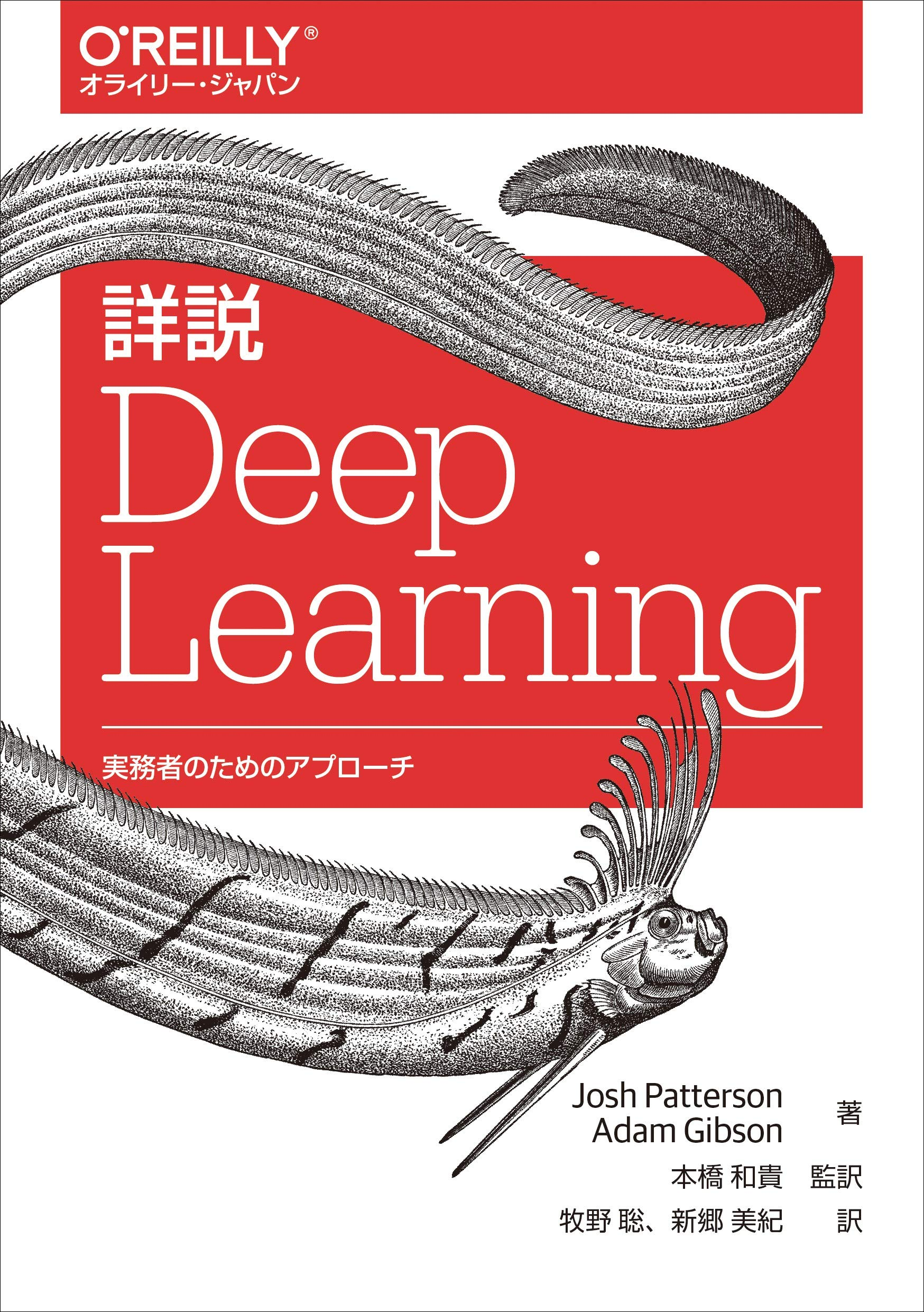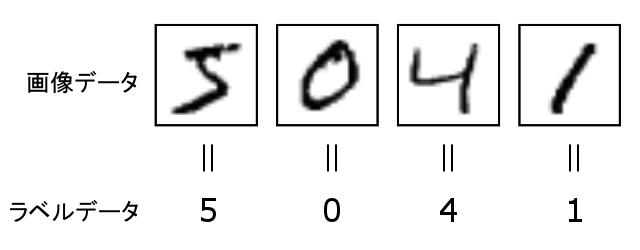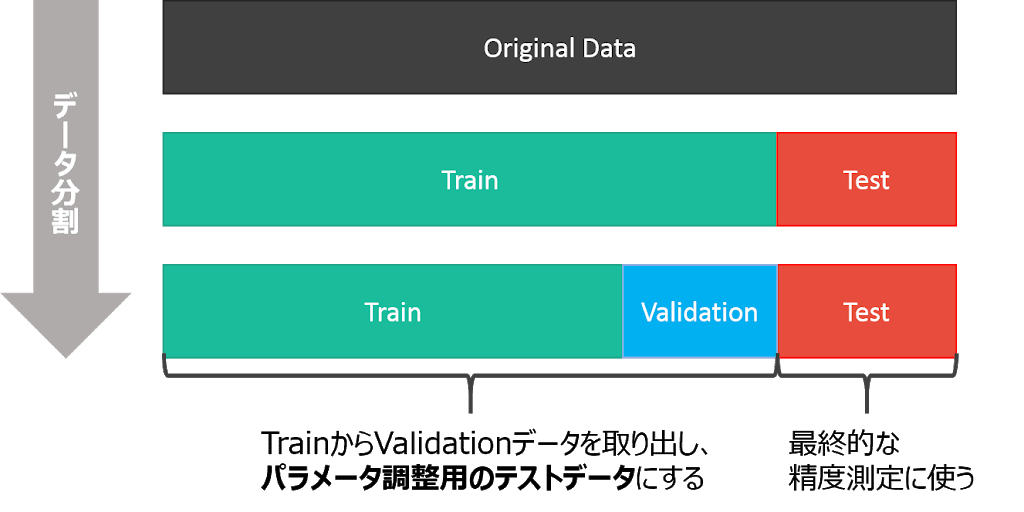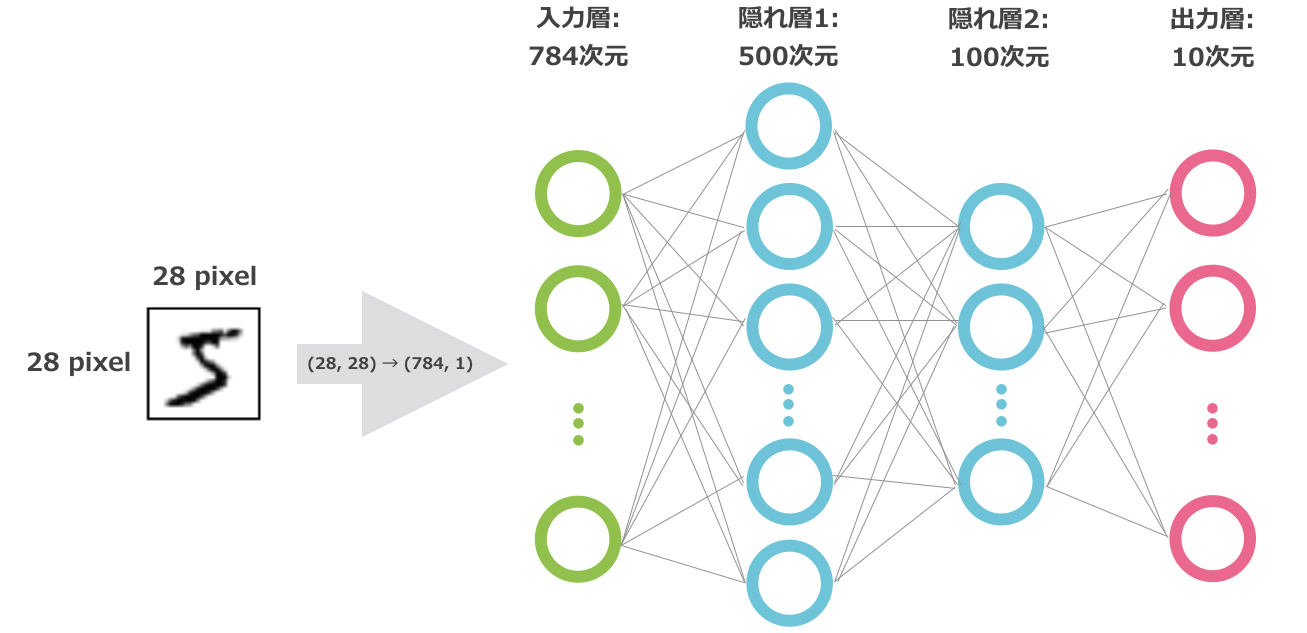# [DL4J] Java deep learning for the first time (handwriting recognition using a fully connected neural network)

Hello. Even though it's Marine Day, Tokyo is still cool.

Today, July 15, 2019, the highest temperature in Tokyo was 25 ° C and the lowest temperature was 19 ° C. According to the records of the Japan Meteorological Agency, the average maximum and minimum temperatures in Tokyo from 1981 to 2010 are as follows.

Average maximum temperature Average minimum temperature
29.0℃ 21.7℃

(Source: https://www.data.jma.go.jp/obd/stats/etrn/view/nml_sfc_d.php?prec_no=44&block_no=47662&year=0month=7&day=1view=p1)

Personally, I like the current climate because it's easy to live in, but how long will it last?

Deeplearning4j / DL4J

By the way, the story changes, and in this article, I will introduce ** Deeplearning4j **, or ** DL4J ** for short, which is being developed by ** Skymind **. As the name implies, DL4J is a deep learning development framework ** that runs in ** JVM languages such as ** Java **, ** Scala **, ** Kotlin **. Other well-known deep learning frameworks include Google's TensorFlow, Keras integrated into it, FaceBook's PyTorch, and Preferred Networks' Chainer. These frameworks are basically assumed to be developed in Python, and you can easily carry out research and development with a small number of codes.

DL4J is differentiated as a ** enterprise framework ** because it can be written in the JVM language that is widely used in corporate systems. ** One of the selling points is that it can be natively linked with big data analysis platforms such as Hadoop and Spark **.

Let's take a look at a sample of building a neural network using DL4J.

# DL4J sample code

The DL4J sample code is abundantly available in the official repositories. https://github.com/deeplearning4j/dl4j-examples

The scale is too big to give it a try, so clone the following repository, which forked only some code this time.

#### `python`

``````
\$ git clone https://github.com/kmotohas/oreilly-book-dl4j-examples-ja
``````

This is the Japanese version of "Deep Learning-A Practitioner's Approach" written by Adam Gibson et al., The author of DL4J itself. Detailed explanation Deep Learning-Approach for practitioners "](https://www.amazon.co.jp/dp/4873118808/) is a public repository of sample code related to this.As a simple example, MLPMnistTwoLayerExample.java recognizes the standard MNIST handwritten numerical data set with a multi-layer perceptron (MLP, also a fully connected neural network in a loose definition). Let's take a look at the contents of -dl4j-examples-ja / blob / master / dl4j-examples / src / main / java / org / deeplearning4j / examples / feedforward / mnist / MLPMNistTwoLayerExample.java).

It is recommended to run the sample using an integrated development environment such as Intellij IDEA, but it is also possible to run it on the command line using a build tool such as Maven.

# Overview of MLPMnistTwoLayerExample.java

The following code is the entire code, omitting the ʻimport` statement at the beginning.

#### `python`

``````
public class MLPMnistTwoLayerExample {

private static Logger log = LoggerFactory.getLogger(MLPMnistSingleLayerExample.class);

public static void main(String[] args) throws Exception {
//number of rows and columns in the input pictures
final int numRows = 28;
final int numColumns = 28;
int outputNum = 10; // number of output classes
int batchSize = 64; // batch size for each epoch
int rngSeed = 123; // random number seed for reproducibility
int numEpochs = 15; // number of epochs to perform
double rate = 0.0015; // learning rate

//Get the DataSetIterators:
DataSetIterator mnistTrain = new MnistDataSetIterator(batchSize, true, rngSeed);
DataSetIterator mnistTest = new MnistDataSetIterator(batchSize, false, rngSeed);

log.info("Build model....");
MultiLayerConfiguration conf = new NeuralNetConfiguration.Builder()
.seed(rngSeed) //include a random seed for reproducibility
.activation(Activation.RELU)
.weightInit(WeightInit.XAVIER)
.updater(new Nesterovs(rate, 0.98)) //specify the rate of change of the learning rate.
.l2(rate * 0.005) // regularize learning model
.list()
.layer(0, new DenseLayer.Builder() //create the first input layer.
.nIn(numRows * numColumns)
.nOut(500)
.build())
.layer(1, new DenseLayer.Builder() //create the second input layer
.nIn(500)
.nOut(100)
.build())
.layer(2, new OutputLayer.Builder(LossFunction.NEGATIVELOGLIKELIHOOD) //create hidden layer
.activation(Activation.SOFTMAX)
.nIn(100)
.nOut(outputNum)
.build())
.build();

MultiLayerNetwork model = new MultiLayerNetwork(conf);
model.init();
model.setListeners(new ScoreIterationListener(5));  //print the score with every iteration

log.info("Train model....");
for( int i=0; i<numEpochs; i++ ){
log.info("Epoch " + i);
model.fit(mnistTrain);
}

log.info("Evaluate model....");
Evaluation eval = new Evaluation(outputNum); //create an evaluation object with 10 possible classes
while(mnistTest.hasNext()){
DataSet next = mnistTest.next();
INDArray output = model.output(next.getFeatures()); //get the networks prediction
eval.eval(next.getLabels(), output); //check the prediction against the true class
}

log.info(eval.stats());
log.info("****************Example finished********************");
}
}
``````

The `main` method of this class is roughly divided into the following four parts.

1. Preparation of `DataSetIterator` --`MultiLayerConfiguration` settings --Building `MultiLayerNetwork` --Training of the constructed neural network model --Performance evaluation of the trained model

I will explain each part in turn.

## 1. Preparation of `DataSetIterator`

Training a model in deep learning is the process of inputting a dataset to the model and updating the parameters to minimize the difference between the expected and actual output.

In DL4J, `DataSetIterator` is used as an iterator to feed data to the model iteratively. -A class called api / src / main / java / org / nd4j / linalg / dataset / api / iterator / DataSetIterator.java) is provided. (Actually [implemented] in the ND4J library, which can also be called the JVM version of Numpy (https://github.com/eclipse/deeplearning4j/blob/master/nd4j/nd4j-backends/nd4j-api-parent/nd4j-api/src/main /java/org/nd4j/linalg/dataset/api/iterator/DataSetIterator.java). It inherits `java.util.Iterator` and` java.io.Serializable`.)

The MNIST dataset of handwritten numbers contains 70,000 handwritten numbers images and correct labels (numbers drawn on the images, 0,1,2,3, ..., 9 information). Generally, these are divided and 60,000 are used as training datasets and 10,000 are used as performance evaluation test datasets.(Source: https://weblabo.oscasierra.net/python/ai-mnist-data-detail.html)

As shown in the figure below, it may be further divided into verification data for hyperparameter tuning such as learning rate, but this time it will not be dealt with.(Source: https://www.procrasist.com/entry/10-cross-validation)

Like other frameworks, DL4J has an iterator dedicated to MNIST. There are also iterators for other well-known datasets such as CIFAR-10 and Tiny ImageNet. See the official documentation (https://deeplearning4j.org/docs/latest/deeplearning4j-nn-iterators) for more information.

Information such as `RecordReaderDataSetIterator` for datasets such as your own images and CSV and` SequenceRecordReaderDataSetIterator` for sequence data is also on the same page.

#### `python`

``````
//Get the DataSetIterators:
DataSetIterator mnistTrain = new MnistDataSetIterator(batchSize, true, rngSeed);
DataSetIterator mnistTest = new MnistDataSetIterator(batchSize, false, rngSeed);
``````

We have an iterator for training and an iterator for testing. [Source code of `MnistDataSetIterator`](https://github.com/eclipse/deeplearning4j/blob/master/deeplearning4j/deeplearning4j-data/deeplearning4j-datasets/src/main/java/org/deeplearning4j/datasets/iterator/impl /MnistDataSetIterator.java), I will quote the constructor used this time.

#### `python`

``````
public MnistDataSetIterator(int batchSize, boolean train, int seed)
``````

The arguments are as follows.

--ʻInt batchSize`: The size of the mini-batch, that is, the number of samples to enter into the model in one iteration of training --`boolean train`: Boolean value indicating whether it is training data or test data --ʻInt seed`: Random seed when shuffling a dataset

## 2. Setting `MultiLayerConfiguration`

This is the part where we are designing the neural network. Use `MultiLayerConfiguration` to stack layers sequentially for Keras.

If you want to build a network with complicated branches, use `ComputationGraphConfiguration`. It's like Keras' functional API. For details, refer to this document.

#### `python`

``````
MultiLayerConfiguration conf = new NeuralNetConfiguration.Builder()
.seed(rngSeed) //include a random seed for reproducibility
.activation(Activation.RELU)
.weightInit(WeightInit.XAVIER)
.updater(new Nesterovs(rate, 0.98)) //specify the rate of change of the learning rate.
.l2(rate * 0.005) // regularize learning model
.list()
.layer(0, new DenseLayer.Builder() //create the first input layer.
.nIn(numRows * numColumns)
.nOut(500)
.build())
.layer(1, new DenseLayer.Builder() //create the second input layer
.nIn(500)
.nOut(100)
.build())
.layer(2, new OutputLayer.Builder(LossFunction.NEGATIVELOGLIKELIHOOD) //create hidden layer
.activation(Activation.SOFTMAX)
.nIn(100)
.nOut(outputNum)
.build())
.build();
``````

`MultiLayerConfiguration` is implemented in the so-called Builder pattern. You can customize your network by specifying parameters in the form `. <Parameter>`.

#### `python`

``````
MultiLayerConfiguration conf = new NeuralNetConfiguration.Builder()
.seed(rngSeed) //include a random seed for reproducibility
.activation(Activation.RELU)
.weightInit(WeightInit.XAVIER)
.updater(new Nesterovs(rate, 0.98)) //specify the rate of change of the learning rate.
.l2(rate * 0.005) // regularize learning model
.list()
``````

The upper half sets the parameters for the entire network. Specifically, the following settings are made.

--Random seed setting with `.seed (rngSeed)` --Set the activation function of each layer to the ReLU function with `.activation (Activation.RELU)` --You can also specify a separate activation function for each layer --Set the initialization method of the neural network weight parameter to "Initialize XAVIER" in `.weightInit (WeightInit.XAVIER)` --Set optimization algorithm (updater) to Nesterovs acceleration method with `.updater (new Nesterovs (rate, 0.98))` --The arguments are learning rate and momentum, respectively. --Set L2 regularization parameters with `.l2 (rate * 0.005)`

#### `python`

``````
.layer(0, new DenseLayer.Builder() //create the first input layer.
.nIn(numRows * numColumns)
.nOut(500)
.build())
.layer(1, new DenseLayer.Builder() //create the second input layer
.nIn(500)
.nOut(100)
.build())
.layer(2, new OutputLayer.Builder(LossFunction.NEGATIVELOGLIKELIHOOD) //create hidden layer
.activation(Activation.SOFTMAX)
.nIn(100)
.nOut(outputNum)
.build())
.build();
``````

The lower half specifies the layer structure of the neural network.

The 0th connection uses a `DenseLayer` with an input of \$ 28 \ times 28 = \$ 784 dimensions and an output of 500 dimensions. The MNIST image has a height of 28 pixels, a width of 28 pixels, and black and white, so the number of channels is 1. In order to input this into the fully connected layer, it is generally necessary to convert from a \$ 28 \ times 28 \$ matrix to a vector. However, this work is unnecessary because it is already recorded flat in the iterator for MNIST of DL4J. The number of 500 dimensions in the output here has no meaning and is a hyperparameter that can be set freely. This number is not always the optimal value.

Similarly, the first connection has a `DenseLayer` with an input of 500 dimensions (the same value as the 0th output) and an output of 100 dimensions. It sounds awkward, but the number 100 dimensions is a value decided by no, and it has no meaning.

The second connection is special and uses ʻOutputLayer`. The input is 100 dimensions as in the previous connection, and the output specifies 10 labels (0 to 9) for the data. The activation function is overwritten with ʻActivation.SOFTMAX`, and` LossFunction.NEGATIVELOGLIKELIHOOD` is set as the loss function. The softmax function is a function used to convert the input value as a probability (a positive value with a sum of 1), and is a set with the Negative Log Likelihood when solving a multiclass classification problem. It is used.

The image of the model set here is as follows.## 3. Build `MultiLayerNetwork`

Create an instance of `MultiLayerNetwork` with` MultiLayerConfiguration` as an argument to create a neural network!

#### `python`

``````
MultiLayerNetwork model = new MultiLayerNetwork(conf);
model.init();
``````

## 4. Training of the constructed neural network model

#### `python`

``````
model.setListeners(new ScoreIterationListener(5));  //print the score with every iteration
for( int i=0; i<numEpochs; i++ ){
log.info("Epoch " + i);
model.fit(mnistTrain);
}
``````

After that, you can train the neural network by calling `fit (DataSetIterator iterator)` of` MultiLayerNetwork` with the iterator of the training data as an argument. The training data is not used only once, but basically repeated multiple times. This repeating unit is called an epoch.

It is also possible to set a listener to monitor the training status. This is an image of Keras Callback. `ScoreIterationListener (int printIterations)` prints the score (loss function value) to the standard output after each iteration (in DL4J terminology, one iteration of the weight parameter is one iteration). ..

Terms around here can be found in the Official Glossary (https://skymind.ai/wiki/glossary). Note that when training a dataset containing 1000 samples with a mini-batch size of 100, 1 epoch is 10 iterations. When training for 30 epochs, it is equivalent to 300 iterations.

You can use `CheckpointListener` when you want to save the model not only at the end of training but also in the middle, or you can use ʻEvaluativeListener` when performing performance evaluation in the middle. For other listeners, see Official Documentation.

## 5. Performance evaluation of the trained model

#### `python`

``````
Evaluation eval = new Evaluation(outputNum); //create an evaluation object with 10 possible classes
while(mnistTest.hasNext()){
DataSet next = mnistTest.next();
INDArray output = model.output(next.getFeatures()); //get the networks prediction
eval.eval(next.getLabels(), output); //check the prediction against the true class
}

log.info(eval.stats());
``````

For model performance evaluation using test data, [`public Evaluation (int num Classes)`](https://github.com/eclipse/deeplearning4j/blob/master/deeplearning4j/deeplearning4j-nn/src/main/java/ Use an instance of org / deeplearning4j / eval / Evaluation.java). Turn the test iterator `mnistTest`, get the vector of the data with the`getFeatures ()`method, and infer the trained model [`public INDArray output (INDArray input) `](https://github.com/ eclipse / deeplearning4j / blob / master / deeplearning4j / deeplearning4j-nn / src / main / java / org / deeplearning4j / nn / multilayer / MultiLayerNetwork.java). Label this inference result and test data [`public void eval (INDArray realOutcomes, INDArray guesses)`](https://github.com/eclipse/deeplearning4j/blob/master/nd4j/nd4j-backends/nd4j-api- parent / nd4j-api / src / main / java / org / nd4j / evaluation / classification / Evaluation.java) When comparing with the method and displaying the result with ʻeval.stats () `, the accuracy / precision / recall / F1 score You can check the value.

1. Preparation of `DataSetIterator` --`MultiLayerConfiguration` settings --Building `MultiLayerNetwork` --Training of the constructed neural network model --Performance evaluation of the trained model